Geometric unary operations on simple feature geometries. These are all generics, with methods for sfg, sfc and sf objects, returning an object of the same class. All operations work on a per-feature basis, ignoring all other features.

## Usage

st_buffer(
x,
dist,
endCapStyle = "ROUND",
joinStyle = "ROUND",
mitreLimit = 1,
singleSide = FALSE,
...
)

st_boundary(x)

st_convex_hull(x)

st_concave_hull(x, ratio, ..., allow_holes)

st_simplify(x, preserveTopology, dTolerance = 0)

st_triangulate(x, dTolerance = 0, bOnlyEdges = FALSE)

st_triangulate_constrained(x)

st_inscribed_circle(x, dTolerance, ...)

st_minimum_rotated_rectangle(x, ...)

st_voronoi(x, envelope, dTolerance = 0, bOnlyEdges = FALSE)

st_polygonize(x)

st_line_merge(x)

st_centroid(x, ..., of_largest_polygon = FALSE)

st_point_on_surface(x)

st_reverse(x)

st_node(x)

st_segmentize(x, dfMaxLength, ...)

## Arguments

x

object of class sfg, sfc or sf

dist

numeric; buffer distance for all, or for each of the elements in x; in case dist is a units object, it should be convertible to arc_degree if x has geographic coordinates, and to st_crs(x)$units otherwise nQuadSegs integer; number of segments per quadrant (fourth of a circle), for all or per-feature; see details endCapStyle character; style of line ends, one of 'ROUND', 'FLAT', 'SQUARE'; see details joinStyle character; style of line joins, one of 'ROUND', 'MITRE', 'BEVEL'; see details mitreLimit numeric; limit of extension for a join if joinStyle 'MITRE' is used (default 1.0, minimum 0.0); see details singleSide logical; if TRUE, single-sided buffers are returned for linear geometries, in which case negative dist values give buffers on the right-hand side, positive on the left; see details ... ignored ratio numeric; fraction convex: 1 returns the convex hulls, 0 maximally concave hulls allow_holes logical; if TRUE, the resulting concave hull may have holes preserveTopology logical; carry out topology preserving simplification? May be specified for each, or for all feature geometries. Note that topology is preserved only for single feature geometries, not for sets of them. If not specified (i.e. the default), then it is internally set equal to FALSE when the input data is specified with projected coordinates or sf_use_s2() returns FALSE. Ignored in all the other cases (with a warning when set equal to FALSE) since the function implicitly calls s2::s2_simplify which always preserve topological relationships (per single feature). dTolerance numeric; tolerance parameter, specified for all or for each feature geometry. If you run st_simplify, the input data is specified with long-lat coordinates and sf_use_s2() returns TRUE, then the value of dTolerance must be specified in meters. bOnlyEdges logical; if TRUE, return lines, else return polygons envelope object of class sfc or sfg containing a POLYGON with the envelope for a voronoi diagram; this only takes effect when it is larger than the default envelope, chosen when envelope is an empty polygon of_largest_polygon logical; for st_centroid: if TRUE, return centroid of the largest (sub)polygon of a MULTIPOLYGON rather than of the whole MULTIPOLYGON dfMaxLength maximum length of a line segment. If x has geographical coordinates (long/lat), dfMaxLength is either a numeric expressed in meter, or an object of class units with length units rad or degree; segmentation in the long/lat case takes place along the great circle, using st_geod_segmentize. ## Value an object of the same class of x, with manipulated geometry. ## Details st_buffer computes a buffer around this geometry/each geometry. If any of endCapStyle, joinStyle, or mitreLimit are set to non-default values ('ROUND', 'ROUND', 1.0 respectively) then the underlying 'buffer with style' GEOS function is used. If a negative buffer returns empty polygons instead of shrinking, set st_use_s2() to FALSE See postgis.net/docs/ST_Buffer.html for details. nQuadSegs, endCapsStyle, joinStyle, mitreLimit and singleSide only work when the GEOS back-end is used: for projected coordinates or when sf_use_s2() is set to FALSE. st_boundary returns the boundary of a geometry st_convex_hull creates the convex hull of a set of points st_concave_hull creates the concave hull of a geometry st_simplify simplifies lines by removing vertices. st_triangulate triangulates set of points (not constrained). st_triangulate requires GEOS version 3.4 or above st_triangulate_constrained returns the constrained delaunay triangulation of polygons; requires GEOS version 3.10 or above st_inscribed_circle returns the maximum inscribed circle for polygon geometries. For st_inscribed_circle, if nQuadSegs is 0 a 2-point LINESTRING is returned with the center point and a boundary point of every circle, otherwise a circle (buffer) is returned where nQuadSegs controls the number of points per quadrant to approximate the circle. st_inscribed_circle requires GEOS version 3.9 or above st_minimum_rotated_rectangle returns the minimum rotated rectangular POLYGON which encloses the input geometry. The rectangle has width equal to the minimum diameter, and a longer length. If the convex hill of the input is degenerate (a line or point) a linestring or point is returned. st_voronoi creates voronoi tesselation. st_voronoi requires GEOS version 3.5 or above st_polygonize creates polygon from lines that form a closed ring. In case of st_polygonize, x must be an object of class LINESTRING or MULTILINESTRING, or an sfc geometry list-column object containing these st_line_merge merges lines. In case of st_line_merge, x must be an object of class MULTILINESTRING, or an sfc geometry list-column object containing these st_centroid gives the centroid of a geometry st_point_on_surface returns a point guaranteed to be on the (multi)surface. st_reverse reverses the nodes in a line st_node adds nodes to linear geometries at intersections without a node, and only works on individual linear geometries st_segmentize adds points to straight lines ## See also chull for a more efficient algorithm for calculating the convex hull ## Examples  ## st_buffer, style options (taken from rgeos gBuffer) l1 = st_as_sfc("LINESTRING(0 0,1 5,4 5,5 2,8 2,9 4,4 6.5)") op = par(mfrow=c(2,3)) plot(st_buffer(l1, dist = 1, endCapStyle="ROUND"), reset = FALSE, main = "endCapStyle: ROUND") plot(l1,col='blue',add=TRUE) plot(st_buffer(l1, dist = 1, endCapStyle="FLAT"), reset = FALSE, main = "endCapStyle: FLAT") plot(l1,col='blue',add=TRUE) plot(st_buffer(l1, dist = 1, endCapStyle="SQUARE"), reset = FALSE, main = "endCapStyle: SQUARE") plot(l1,col='blue',add=TRUE) plot(st_buffer(l1, dist = 1, nQuadSegs=1), reset = FALSE, main = "nQuadSegs: 1") plot(l1,col='blue',add=TRUE) plot(st_buffer(l1, dist = 1, nQuadSegs=2), reset = FALSE, main = "nQuadSegs: 2") plot(l1,col='blue',add=TRUE) plot(st_buffer(l1, dist = 1, nQuadSegs= 5), reset = FALSE, main = "nQuadSegs: 5") plot(l1,col='blue',add=TRUE)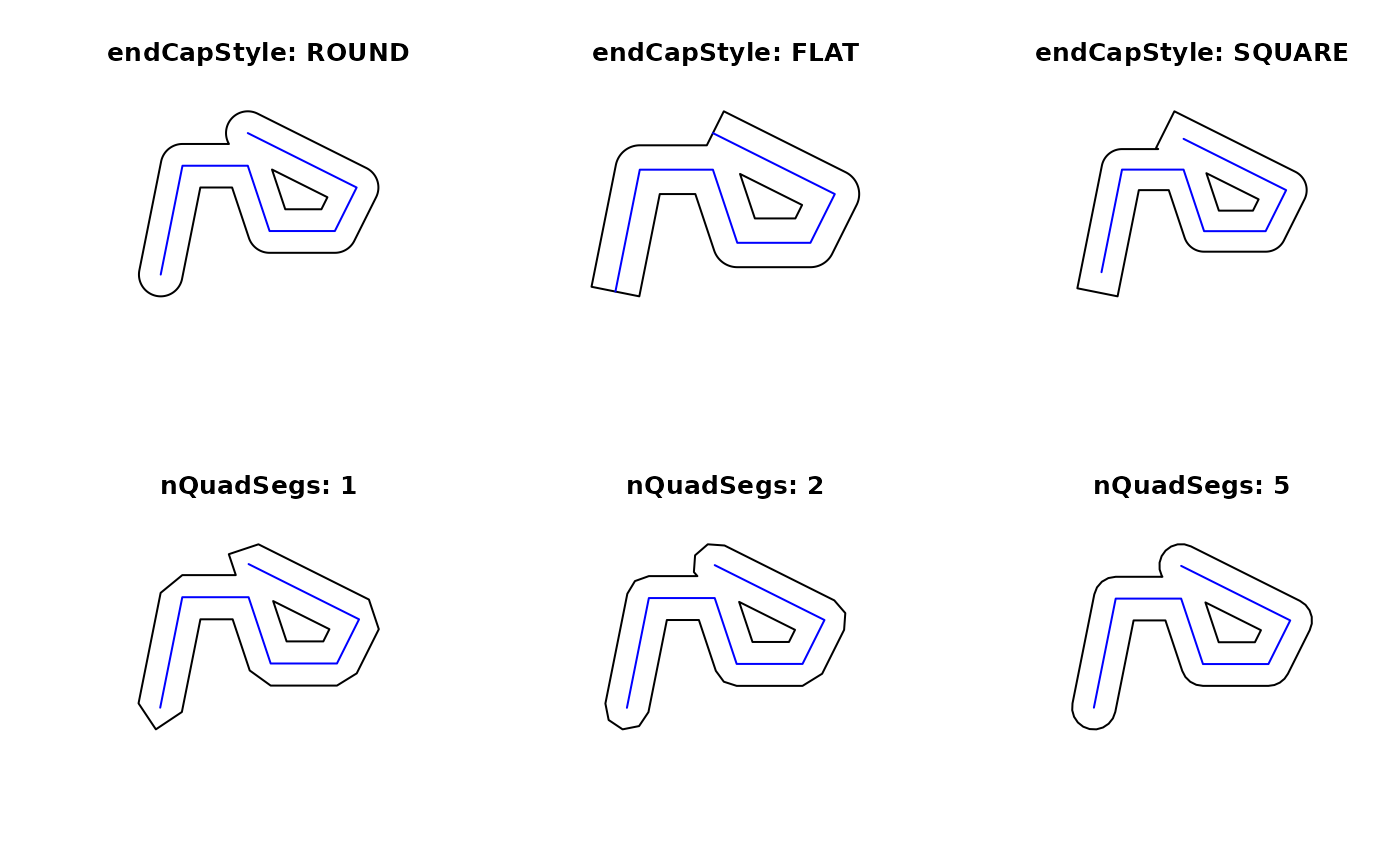par(op) l2 = st_as_sfc("LINESTRING(0 0,1 5,3 2)") op = par(mfrow = c(2, 3)) plot(st_buffer(l2, dist = 1, joinStyle="ROUND"), reset = FALSE, main = "joinStyle: ROUND") plot(l2, col = 'blue', add = TRUE) plot(st_buffer(l2, dist = 1, joinStyle="MITRE"), reset = FALSE, main = "joinStyle: MITRE") plot(l2, col= 'blue', add = TRUE) plot(st_buffer(l2, dist = 1, joinStyle="BEVEL"), reset = FALSE, main = "joinStyle: BEVEL") plot(l2, col= 'blue', add=TRUE) plot(st_buffer(l2, dist = 1, joinStyle="MITRE" , mitreLimit=0.5), reset = FALSE, main = "mitreLimit: 0.5") plot(l2, col = 'blue', add = TRUE) plot(st_buffer(l2, dist = 1, joinStyle="MITRE",mitreLimit=1), reset = FALSE, main = "mitreLimit: 1") plot(l2, col = 'blue', add = TRUE) plot(st_buffer(l2, dist = 1, joinStyle="MITRE",mitreLimit=3), reset = FALSE, main = "mitreLimit: 3") plot(l2, col = 'blue', add = TRUE)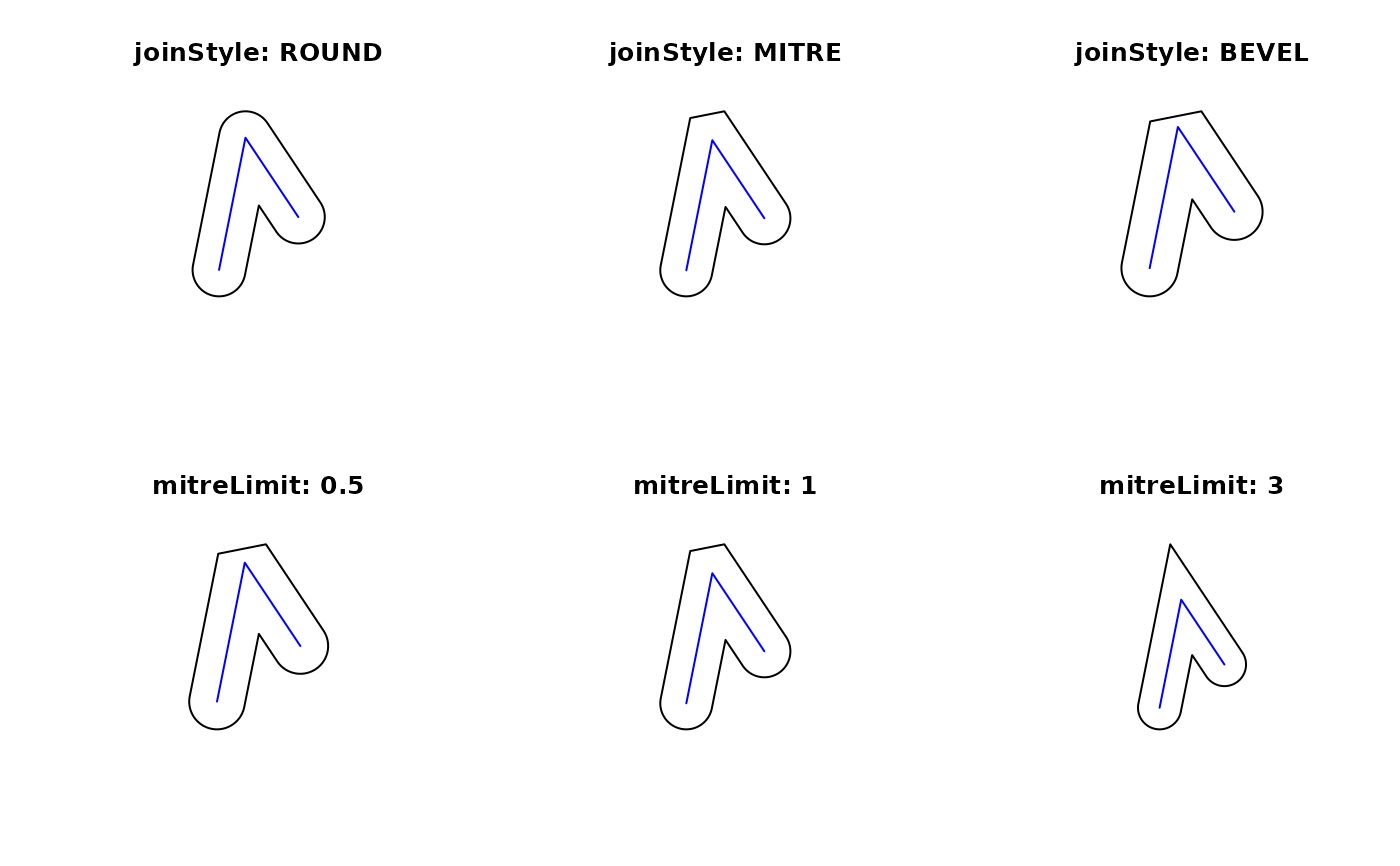par(op) nc = st_read(system.file("shape/nc.shp", package="sf")) #> Reading layer nc' from data source #> /home/runner/work/_temp/Library/sf/shape/nc.shp' using driver ESRI Shapefile' #> Simple feature collection with 100 features and 14 fields #> Geometry type: MULTIPOLYGON #> Dimension: XY #> Bounding box: xmin: -84.32385 ymin: 33.88199 xmax: -75.45698 ymax: 36.58965 #> Geodetic CRS: NAD27 nc_g = st_geometry(nc) plot(st_convex_hull(nc_g)) plot(nc_g, border = grey(.5), add = TRUE)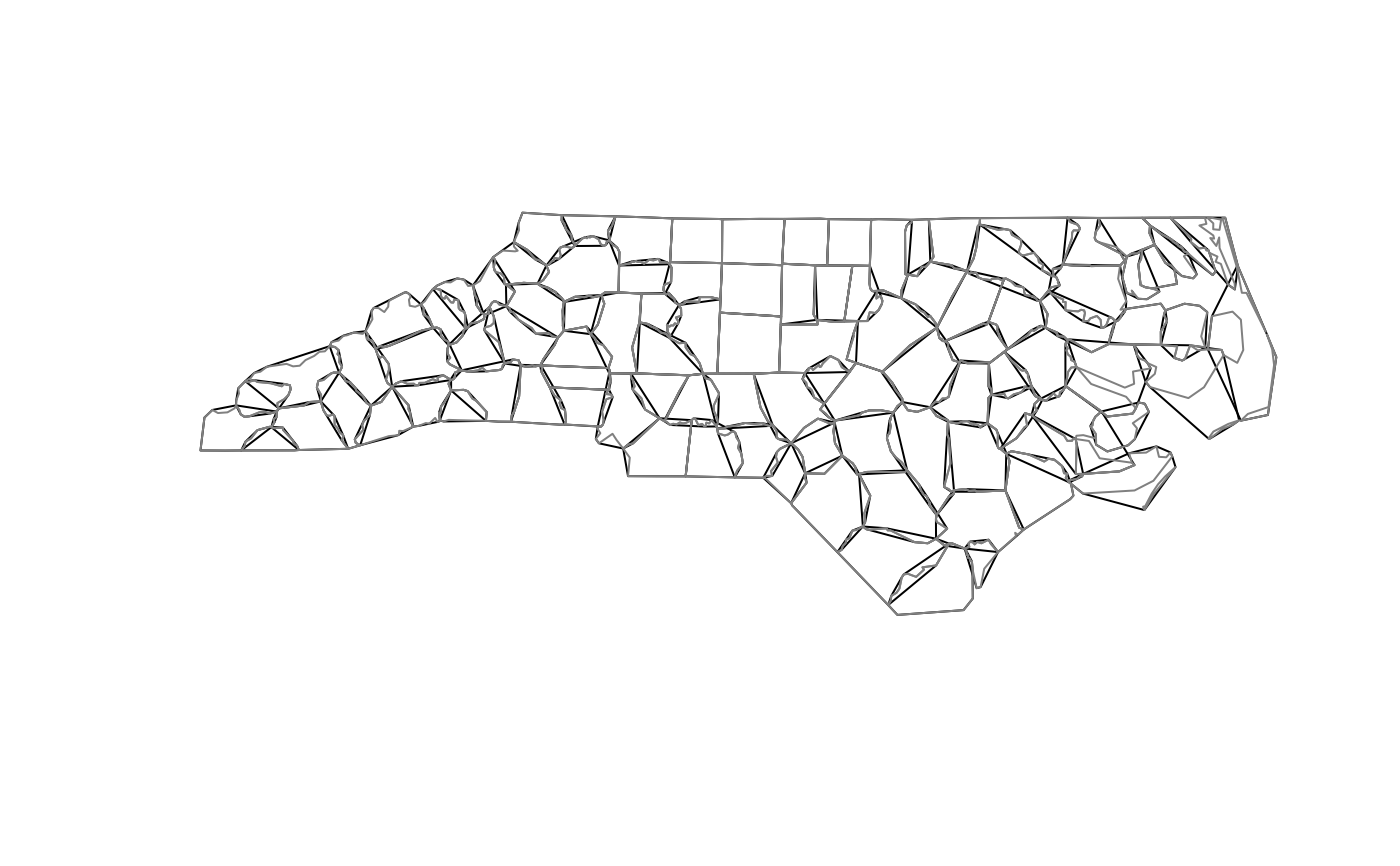set.seed(131) if (compareVersion(sf_extSoftVersion()[["GEOS"]], "3.11.0") > -1) { pts = cbind(runif(100), runif(100)) m = st_multipoint(pts) co = sf:::st_concave_hull(m, 0.3) coh = sf:::st_concave_hull(m, 0.3, allow_holes = TRUE) plot(co, col = 'grey') plot(coh, add = TRUE, border = 'red') plot(m, add = TRUE) } # st_simplify examples: op = par(mfrow = c(2, 3), mar = rep(0, 4)) plot(nc_g) plot(st_simplify(nc_g, dTolerance = 1e3)) # 1000m plot(st_simplify(nc_g, dTolerance = 5e3)) # 5000m nc_g_planar = st_transform(nc_g, 2264) # planar coordinates, US foot plot(nc_g_planar) plot(st_simplify(nc_g_planar, dTolerance = 1e3)) # 1000 foot plot(st_simplify(nc_g_planar, dTolerance = 5e3)) # 5000 foot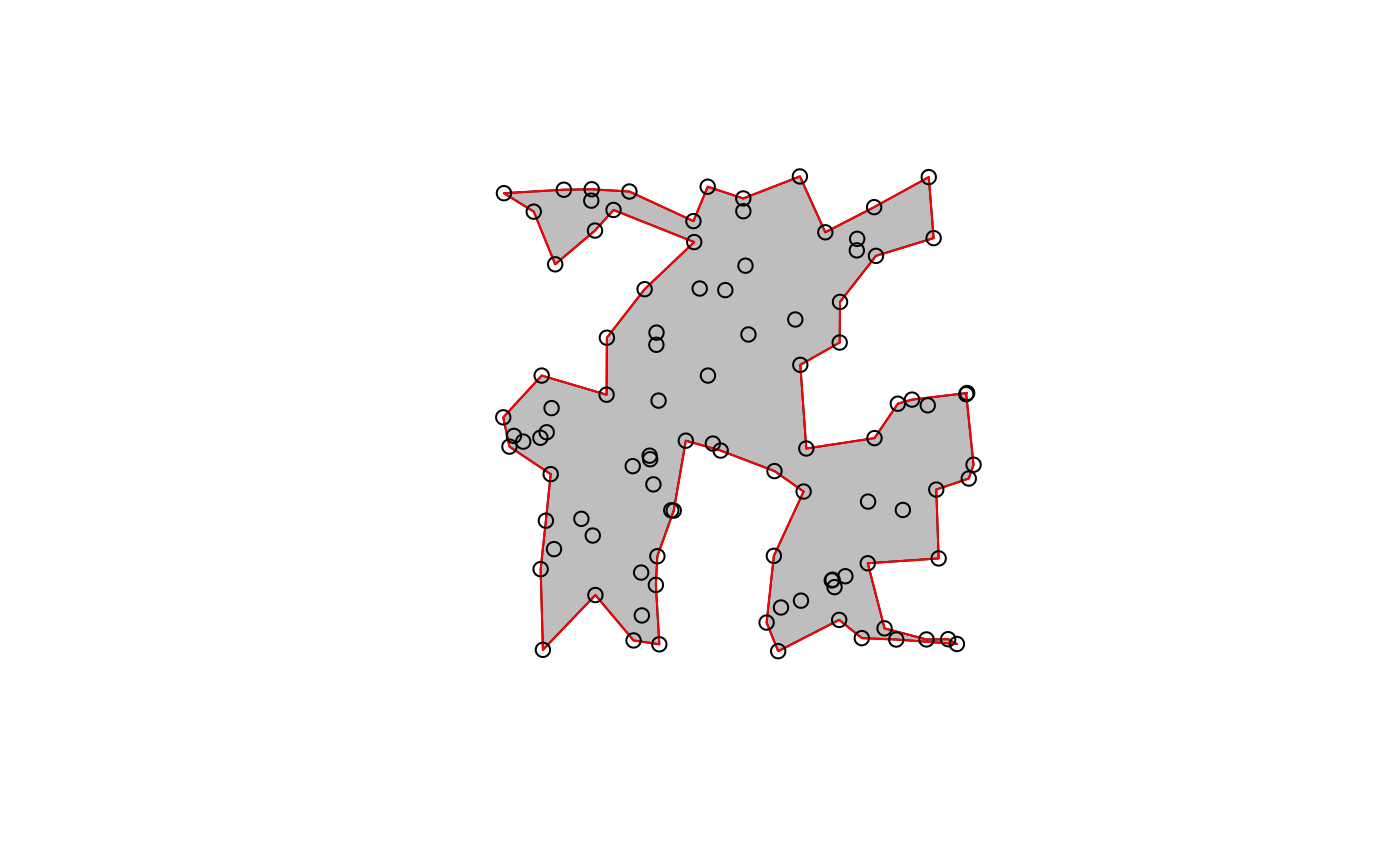par(op) if (compareVersion(sf_extSoftVersion()[["GEOS"]], "3.10.0") > -1) { pts = rbind(c(0,0), c(1,0), c(1,1), c(.5,.5), c(0,1), c(0,0)) po = st_polygon(list(pts)) co = st_triangulate_constrained(po) tr = st_triangulate(po) plot(po, col = NA, border = 'grey', lwd = 15) plot(tr, border = 'green', col = NA, lwd = 5, add = TRUE) plot(co, border = 'red', col = 'NA', add = TRUE) }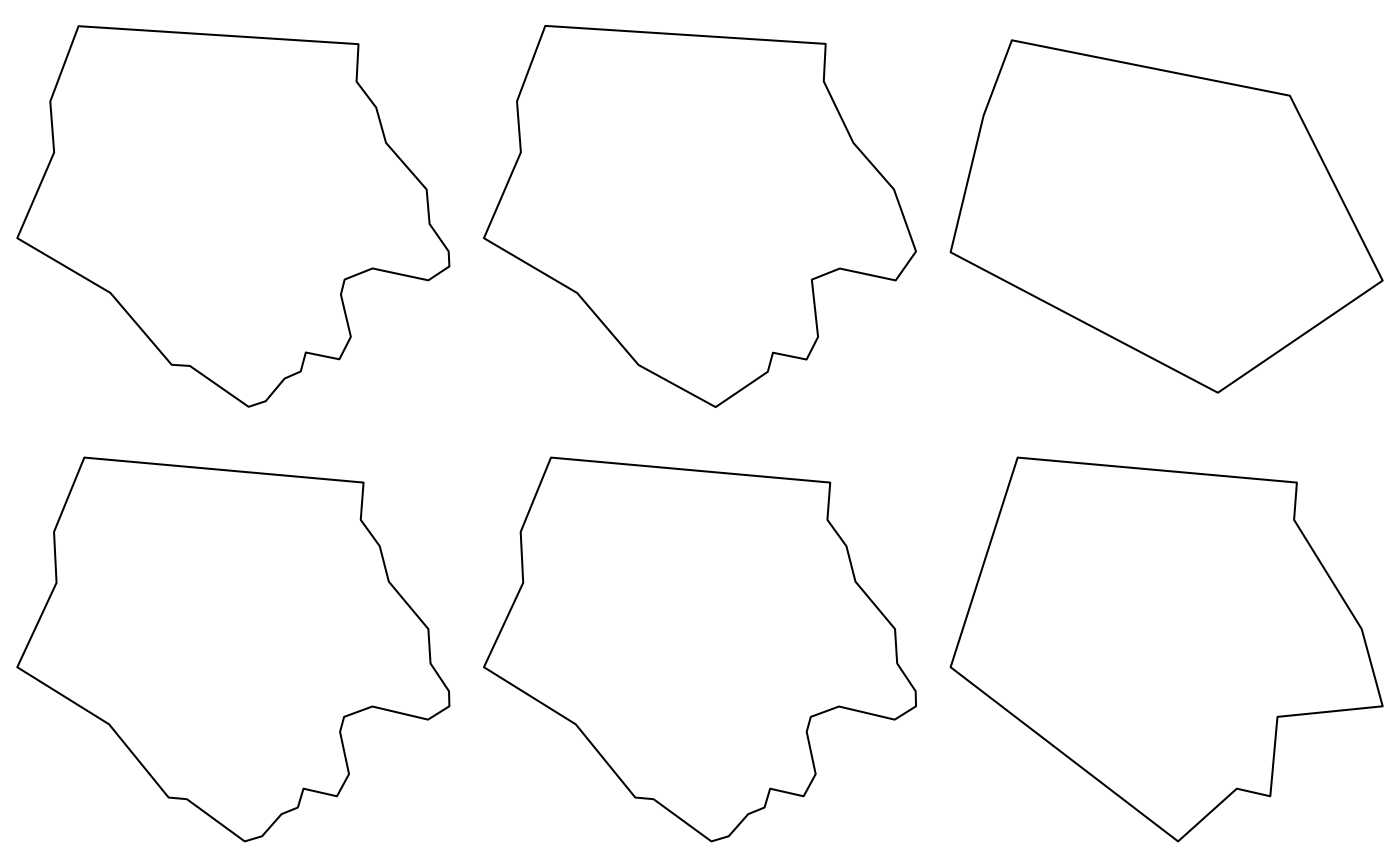if (compareVersion(sf_extSoftVersion()[["GEOS"]], "3.9.0") > -1) { nc_t = st_transform(nc, 'EPSG:2264') x = st_inscribed_circle(st_geometry(nc_t)) plot(st_geometry(nc_t), asp = 1, col = grey(.9)) plot(x, add = TRUE, col = '#ff9999') }set.seed(1) x = st_multipoint(matrix(runif(10),,2)) box = st_polygon(list(rbind(c(0,0),c(1,0),c(1,1),c(0,1),c(0,0)))) if (compareVersion(sf_extSoftVersion()[["GEOS"]], "3.5.0") > -1) { v = st_sfc(st_voronoi(x, st_sfc(box))) plot(v, col = 0, border = 1, axes = TRUE) plot(box, add = TRUE, col = 0, border = 1) # a larger box is returned, as documented plot(x, add = TRUE, col = 'red', cex=2, pch=16) plot(st_intersection(st_cast(v), box)) # clip to smaller box plot(x, add = TRUE, col = 'red', cex=2, pch=16) # matching Voronoi polygons to data points: # https://github.com/r-spatial/sf/issues/1030 # generate 50 random unif points: n = 100 pts = st_as_sf(data.frame(matrix(runif(n), , 2), id = 1:(n/2)), coords = c("X1", "X2")) # compute Voronoi polygons: pols = st_collection_extract(st_voronoi(do.call(c, st_geometry(pts)))) # match them to points: pts$pols = pols[unlist(st_intersects(pts, pols))]
plot(pts["id"], pch = 16) # ID is color
plot(st_set_geometry(pts, "pols")["id"], xlim = c(0,1), ylim = c(0,1), reset = FALSE)
layout(matrix(1)) # reset plot layout
}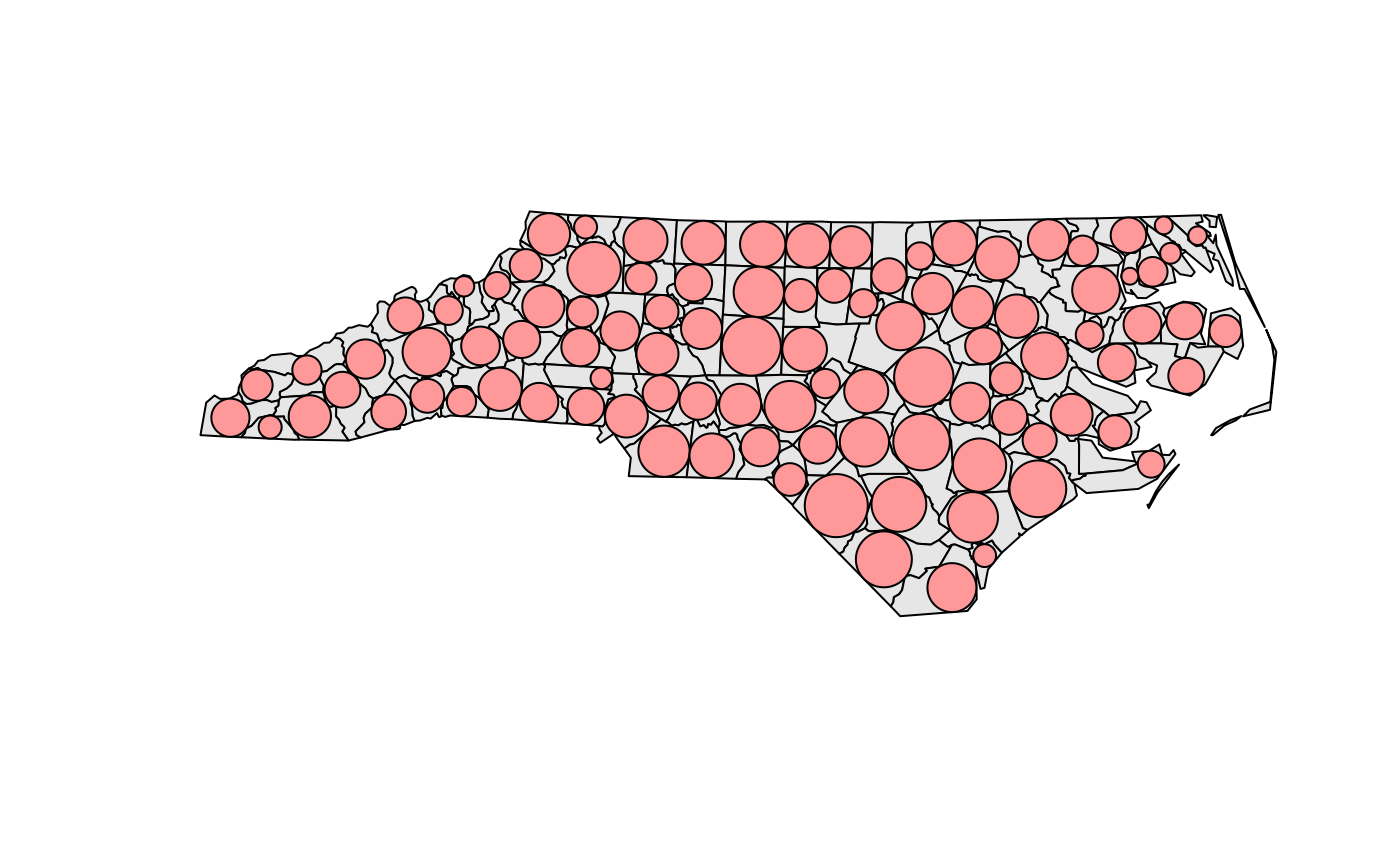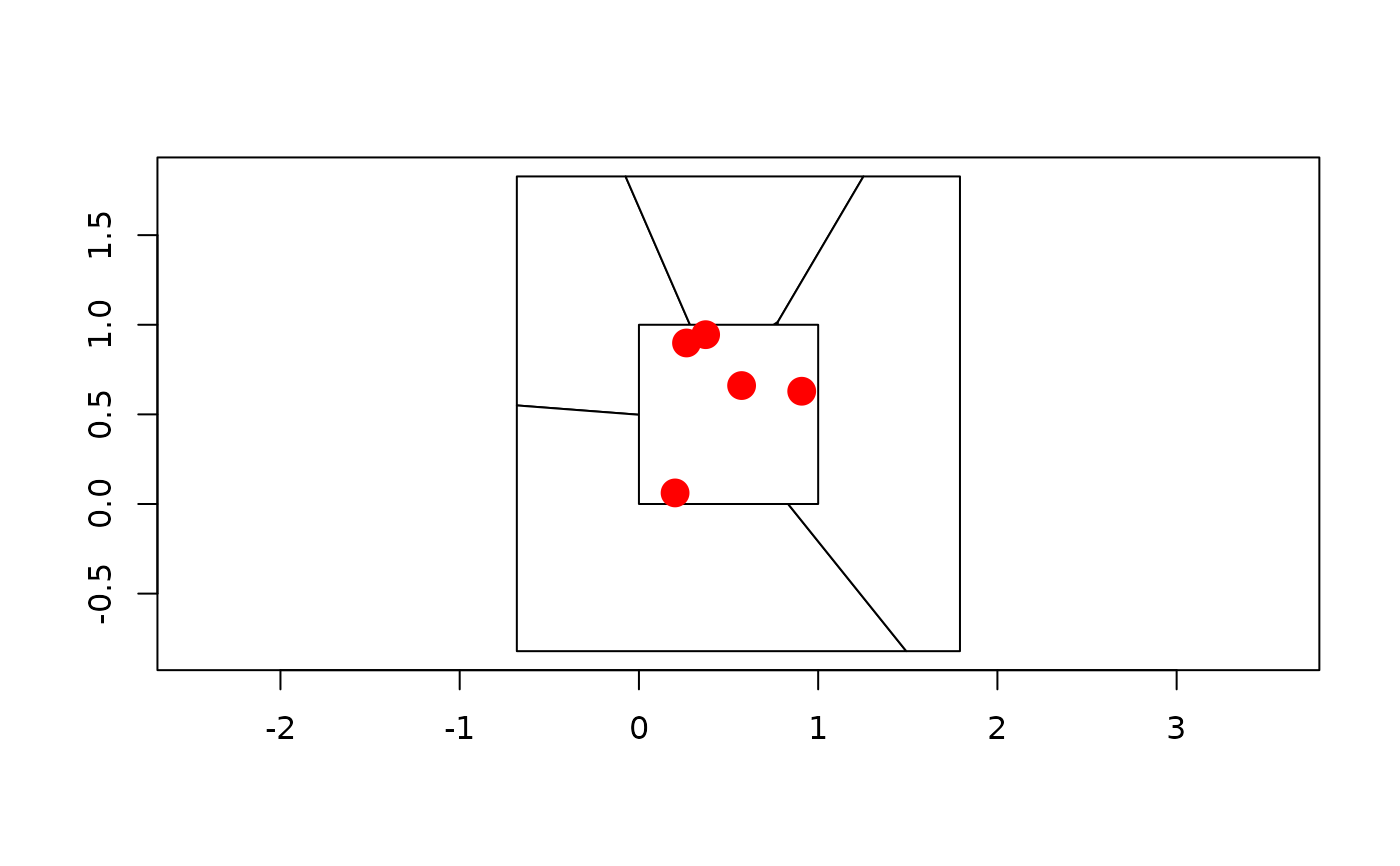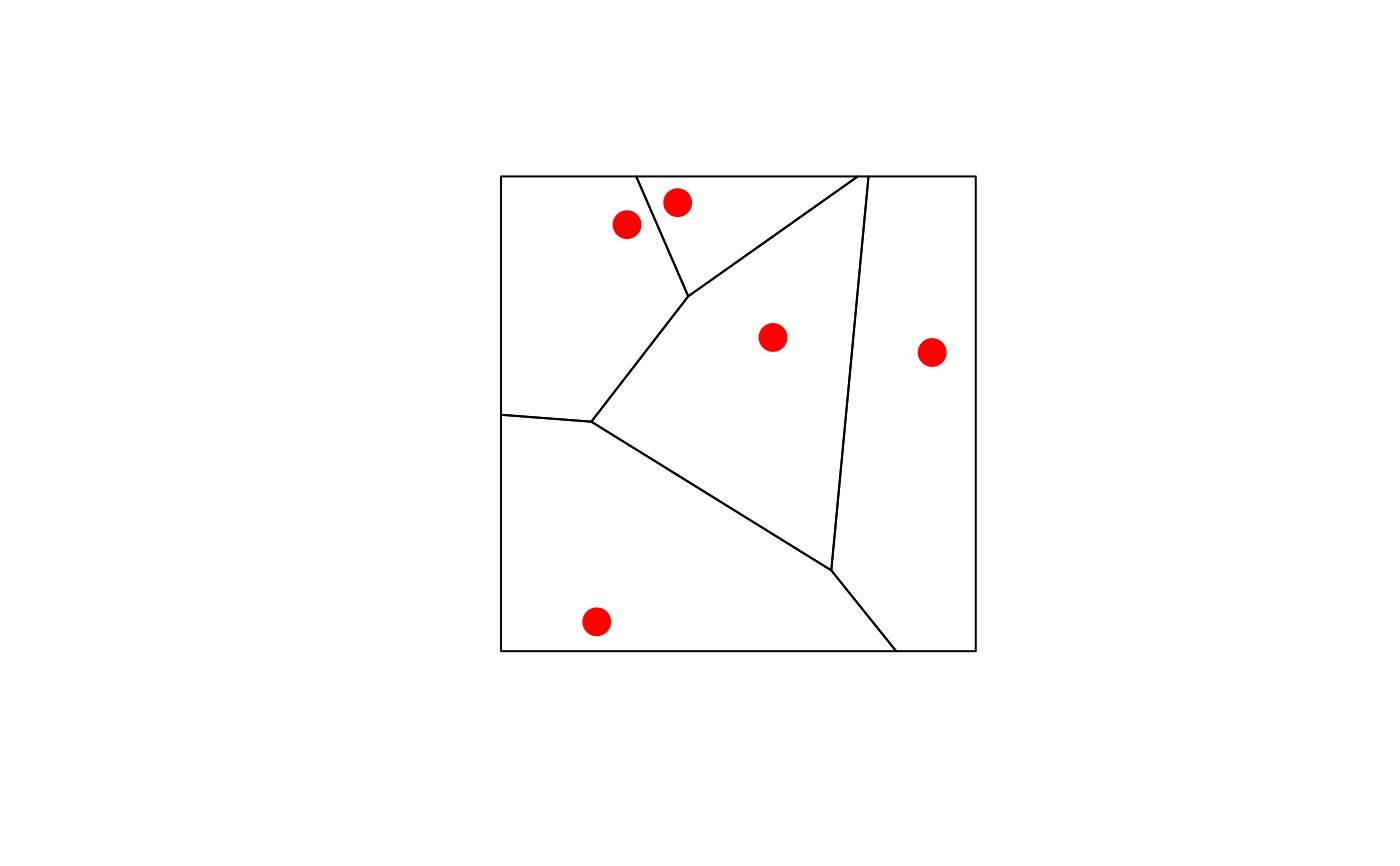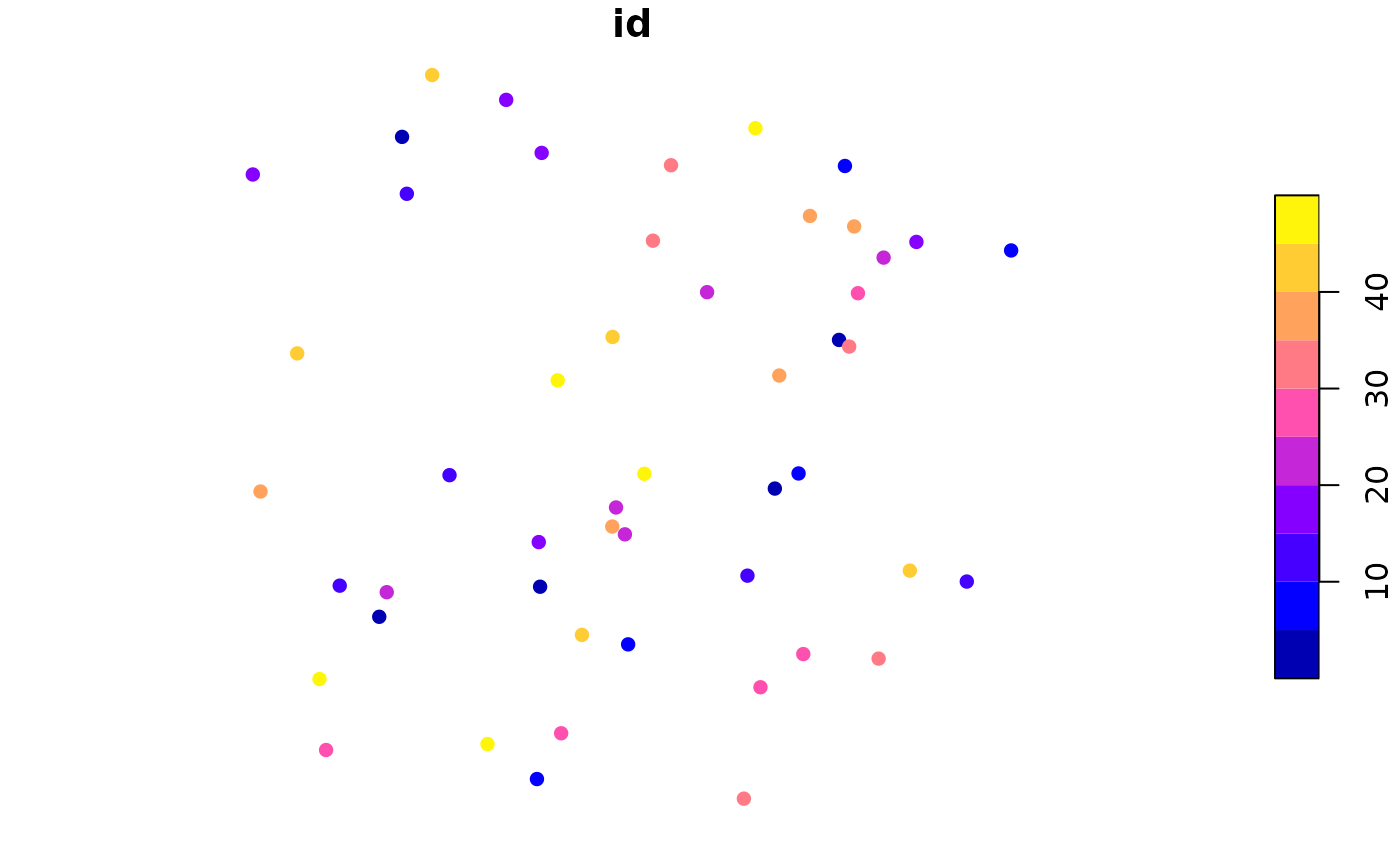mls = st_multilinestring(list(matrix(c(0,0,0,1,1,1,0,0),,2,byrow=TRUE)))
st_polygonize(st_sfc(mls))
#> Geometry set for 1 feature
#> Geometry type: GEOMETRYCOLLECTION
#> Dimension:     XY
#> Bounding box:  xmin: 0 ymin: 0 xmax: 1 ymax: 1
#> CRS:           NA
#> GEOMETRYCOLLECTION (POLYGON ((0 0, 0 1, 1 1, 0 ...
mls = st_multilinestring(list(rbind(c(0,0), c(1,1)), rbind(c(2,0), c(1,1))))
st_line_merge(st_sfc(mls))
#> Geometry set for 1 feature
#> Geometry type: LINESTRING
#> Dimension:     XY
#> Bounding box:  xmin: 0 ymin: 0 xmax: 2 ymax: 1
#> CRS:           NA
#> LINESTRING (0 0, 1 1, 2 0)
plot(nc_g, axes = TRUE)
plot(st_centroid(nc_g), add = TRUE, pch = 3, col = 'red')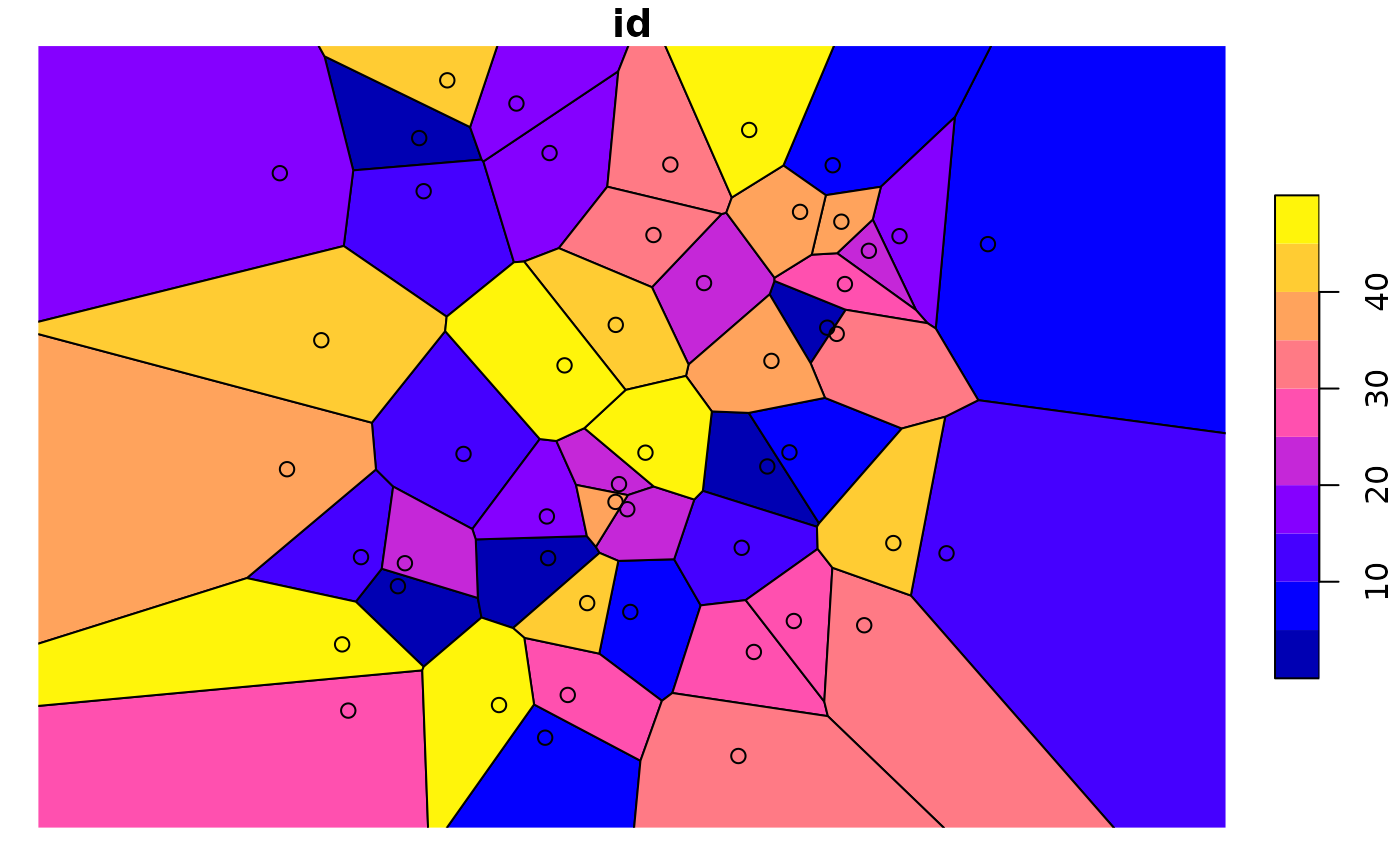mp = st_combine(st_buffer(st_sfc(lapply(1:3, function(x) st_point(c(x,x)))), 0.2 * 1:3))
plot(mp)
plot(st_centroid(mp), add = TRUE, col = 'red') # centroid of combined geometry
plot(st_centroid(mp, of_largest_polygon = TRUE), add = TRUE, col = 'blue', pch = 3)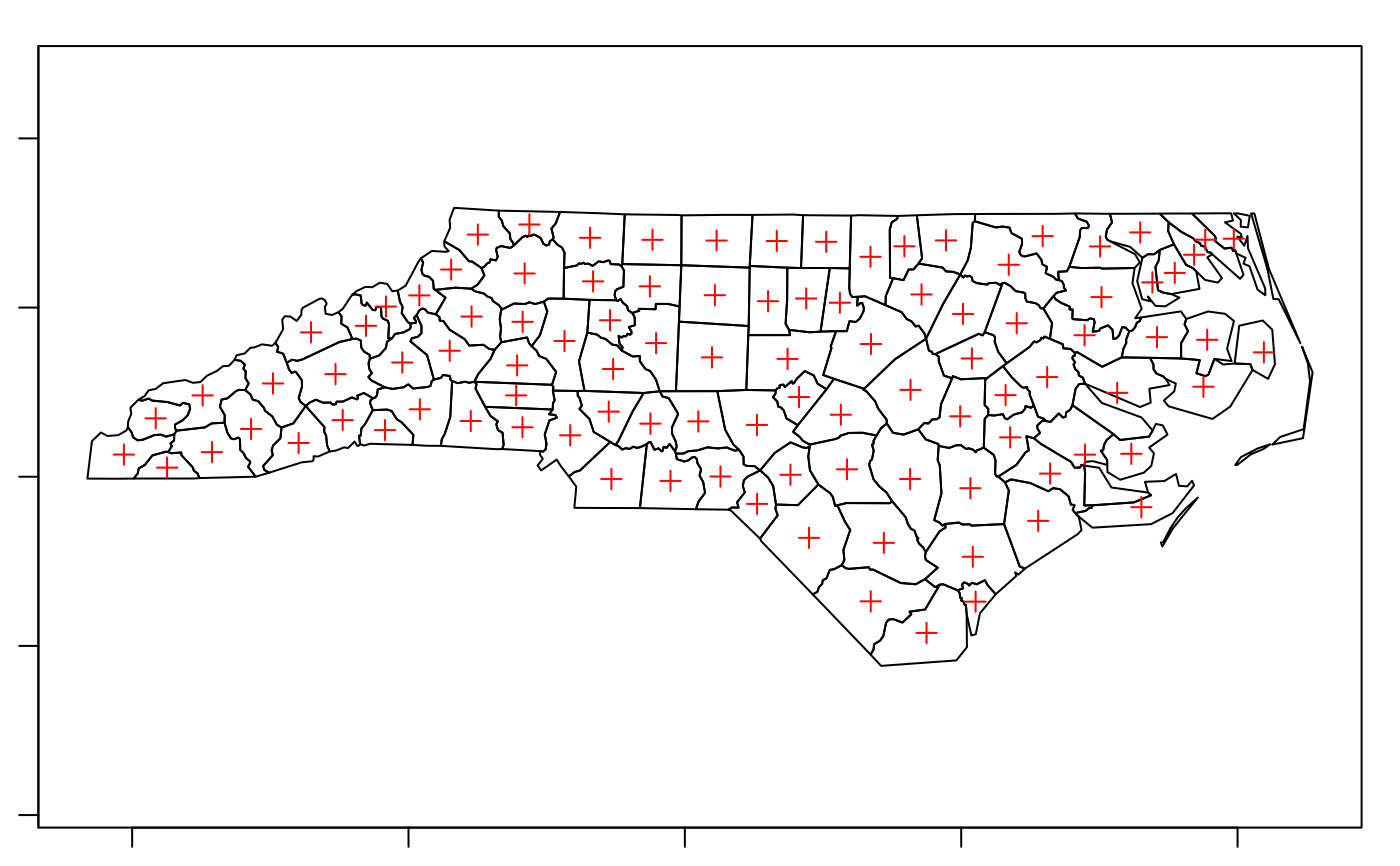plot(nc_g, axes = TRUE)
plot(st_point_on_surface(nc_g), add = TRUE, pch = 3, col = 'red')
#> Warning: st_point_on_surface may not give correct results for longitude/latitude data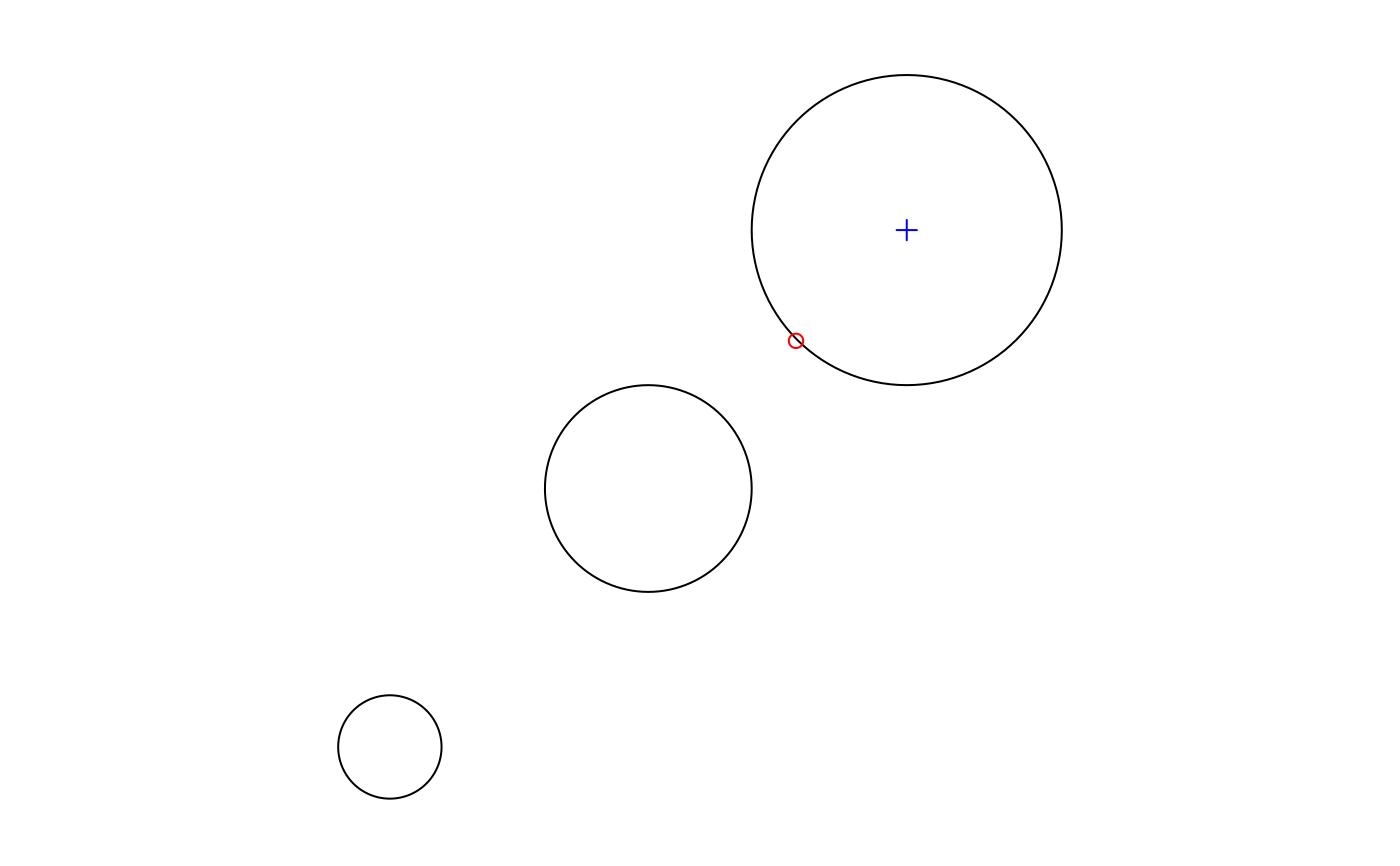if (compareVersion(sf_extSoftVersion()[["GEOS"]], "3.7.0") > -1) {
st_reverse(st_linestring(rbind(c(1,1), c(2,2), c(3,3))))
}
#> LINESTRING (3 3, 2 2, 1 1)
(l = st_linestring(rbind(c(0,0), c(1,1), c(0,1), c(1,0), c(0,0))))
#> LINESTRING (0 0, 1 1, 0 1, 1 0, 0 0)
st_polygonize(st_node(l))
#> GEOMETRYCOLLECTION (POLYGON ((0 0, 0.5 0.5, 1 0, 0 0)), POLYGON ((0.5 0.5, 0 1, 1 1, 0.5 0.5)))
st_node(st_multilinestring(list(rbind(c(0,0), c(1,1), c(0,1), c(1,0), c(0,0)))))
#> MULTILINESTRING ((0 0, 0.5 0.5), (0.5 0.5, 1 1, 0 1, 0.5 0.5), (0.5 0.5, 1 0, 0 0))
sf = st_sf(a=1, geom=st_sfc(st_linestring(rbind(c(0,0),c(1,1)))), crs = 4326)
if (require(lwgeom, quietly = TRUE)) {
seg = st_segmentize(sf, units::set_units(100, km))
`